### SECOND Function – Examples in Excel, VBA, Google Sheets

This tutorial demonstrates how to use the Excel SECOND Function in Excel to get the seconds from a time.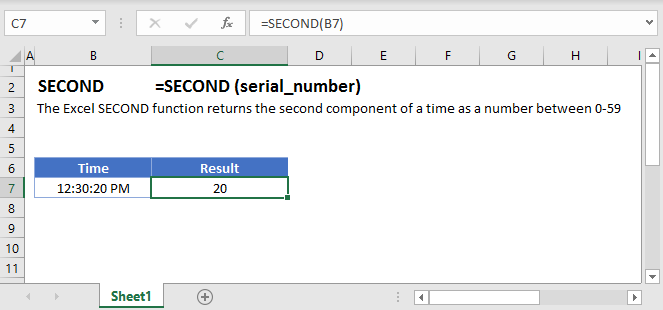## SECOND Function Overview:

The SECOND Function Returns the second as a number (0-59)

To use the SECOND Excel Worksheet Function, Select cell and Type: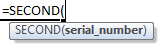(Notice how the formula input appear)

## SECOND Function syntax and Inputs:

serial_number – The time, represented as a fraction of the day or entered as time surrounded by quotations (“s”). Example: You can not enter 8:00:00 pm directly into the cell. Instead you would need to use the corresponding fraction of the day: .3333 or the time surrounded by quorations: “8:00:00 pm”. Alternatively, you can reference a cell with the time entered. Excel automatically converts times stored in cells into a fractional number (unless the time is stored as text).

AutoMacro - VBA Code Generator

## SECOND Examples

The SECOND Function returns the second number of a time: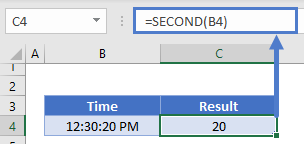### SECOND and TIME Functions

Using the TIME and SECOND Functions you can create a new time with the second number from the original time: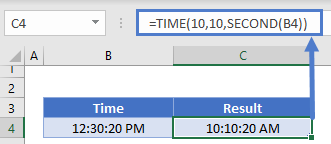## SECOND in Google Sheets

The SECOND Function works exactly the same in Google Sheets as in Excel: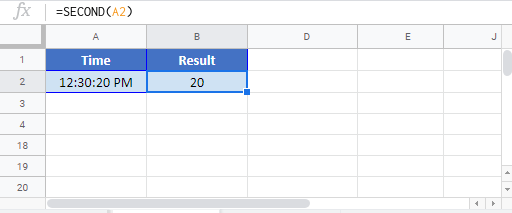## SECOND Examples in VBA

You can also use the SECOND function in VBA. Type:
`application.worksheetfunction.second(serial_number)`
For the function arguments (serial_number, etc.), you can either enter them directly into the function, or define variables to use instead.

Return to the List of all Functions in Excel

## Excel Practice Worksheet

Practice Excel functions and formulas with our 100% free practice worksheets!

• Automatically Graded Exercises
• Learn Excel, Inside Excel!